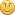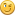## warp, but not everywhere

Started by Dune, July 22, 2016, 07:35:12 am

#### Dune

#####July 22, 2016, 07:35:12 am
I can't get my head around something. I have a simple shape line (road), want it warped (alongside a river), but in a certain area (masked by soft simple shape) it shouldn't be warped, but straight. The localized straight SSS-line should gradually blend into the warped line. I don't want to (and can't) use a painted mask.

The yellow box is supposed to be soft area for the straight line.

If anyone can solve this, I'd be eternally grateful.

#### AP

#####July 22, 2016, 07:49:50 am #1
I did a quick test but it seems difficult to have it blend from the straight to the warped parts.

#### Hetzen

#####July 22, 2016, 07:55:32 am #2 Last Edit: July 22, 2016, 08:08:45 am by Hetzen
If you can get a scaler value out of the displacement warping your SSS road, then use another SSS where your yellow box is, compliment the output (so the yellow area is black) and multiply this with your displacement scaler value, which then goes into a displacement shader back into your warper.

Does that make sense? Should work.

Probably Smooth Step on the edge profile will make a better blend.

`<terragen_clip> <surface_layer name = "Surface layer 01" gui_use_node_pos = "1" gui_node_pos = "-1680 340 0" gui_group = "" enable = "1" input_node = "" gui_use_preview_patch_size = "0" gui_preview_patch_size = "1000 1000" apply_colour = "1" diffuse_colour = "0.5 0.5 0.5" colour_function = "" enable_test_colour = "1" test_colour = "1 0 1" luminous = "0" luminosity_multiplier = "1" luminosity_tint = "1 1 1" luminosity_function = "" displacement_direction = "1" displacement_multiplier = "1" displacement_function = "" displacement_offset = "0" smoothing_effect = "0" smoothing_amount = "1" child_layers = "" coverage = "1" fractal_breakup = "0" breakup_shader = "" fractal_contrast = "0" invert_breakup = "0" only_breakup_colour = "0" blend_by_shader = "1" blending_shader = "Warp input shader 01" invert_blendshader = "0" blend_as_coverage = "1" limit_maximum_altitude = "0" maximum_altitude = "1000" max_alt_fuzzy_zone = "200" limit_minimum_altitude = "0" minimum_altitude = "200" min_alt_fuzzy_zone = "200" altitude_key = "1" use_Y_for_altitude = "0" limit_maximum_slope = "0" maximum_slope_angle = "60" max_slope_fuzzy_zone = "20" limit_minimum_slope = "0" minimum_slope_angle = "30" min_slope_fuzzy_zone = "10" slope_key = "0" use_Y_for_slope = "0" intersect_underlying = "0" intersection_mode = "0" intersection_zone = "2" smoothing_scale = "0" intersection_shift = "2" min_intersection_shift = "-2" fuzzy_zone_softness = "1" > </surface_layer> <displacement_shader_to_scalar name = "Displacement shader to scalar 01" gui_use_node_pos = "1" gui_node_pos = "-1520 740 0" gui_group = "" enable = "0" input_node = "Power fractal shader v3 01" gui_use_preview_patch_size = "0" gui_preview_patch_size = "1000 1000" > </displacement_shader_to_scalar> <power_fractal_shader_v3 name = "Power fractal shader v3 01" gui_use_node_pos = "1" gui_node_pos = "-1520 800 0" gui_group = "" enable = "1" input_node = "" gui_use_preview_patch_size = "0" gui_preview_patch_size = "1000 1000" seed = "25706" feature_scale = "15" lead-in_scale = "100" smallest_scale = "10" noise_octaves = "5" noise_stretch_XYZ = "1 1 1" apply_high_colour = "1" high_colour = "1 1 1" apply_low_colour = "1" low_colour = "0 0 0" colour_contrast = "0.5" colour_offset = "-0.575" colour_roughness = "0" clamp_high_colour = "0" clamp_low_colour = "0" apply_displacement = "1" displacement_direction = "1" displacement_amplitude = "1" displacement_offset = "0" displacement_roughness = "1" displacement_spike_limit = "1" continue_spike_limit = "0" adjust_coastline = "0" coastline_altitude = "0" coastline_smoothing = "30" noise_flavour = "0" ridge_smoothing = "0.1" gully_smoothing = "0.1" noise_variation = "1" variation_method = "2" buoyancy_from_variation = "0.25" clumping_of_variation = "0.25" distort_by_normal = "0" distortion_by_normal = "5" lead-in_warp_effect = "0" lead-in_warp_amount = "0.5" less_warp_at_feature_scale = "0" allow_vertical_warp = "0" four-d_noise = "0" four-d_noise_speed = "0.1" reference_frame_number = "0" blend_by_shader = "0" blending_shader = "" fit_blendshader_to_this = "0" invert_blendshader = "0" > </power_fractal_shader_v3> <simple_shape_shader name = "Yellow Box Area SSS" gui_use_node_pos = "1" gui_node_pos = "-1320 800 0" gui_group = "" enable = "1" input_node = "" gui_use_preview_patch_size = "0" gui_preview_patch_size = "1000 1000" type_of_shape = "0" position = "0 0 0" size = "200 100" rotation = "0" polygon_sides = "5" draw_shape_edges_in_preview = "1" apply_colour = "1" apply_main_colour = "1" colour = "1 1 1" apply_edge_colour = "1" edge_colour = "0 0 0" colour_edge_profile = "1" colour_edge_width = "20" colour_edge_units = "0" colour_position_key = "1" apply_displacement = "0" displace_relative_to_surface = "1" displace_relative_to_shader_position = "0" displacement_direction = "1" displacement_amplitude = "1" displacement_offset = "0" displacement_edge_profile = "0" displacement_edge_width = "50" displacement_edge_units = "0" displacement_position_key = "1" > </simple_shape_shader> <complement_scalar name = "Complement scalar 01" gui_use_node_pos = "1" gui_node_pos = "-1320 740 0" gui_group = "" enable = "1" input_node = "Yellow Box Area SSS" gui_use_preview_patch_size = "0" gui_preview_patch_size = "1000 1000" > </complement_scalar> <simple_shape_shader name = "Road SSS" gui_use_node_pos = "1" gui_node_pos = "-1740 740 0" gui_group = "" enable = "1" input_node = "" gui_use_preview_patch_size = "0" gui_preview_patch_size = "1000 1000" type_of_shape = "0" position = "0 0 0" size = "5 1000" rotation = "0" polygon_sides = "5" draw_shape_edges_in_preview = "1" apply_colour = "1" apply_main_colour = "1" colour = "1 1 1" apply_edge_colour = "1" edge_colour = "0 0 0" colour_edge_profile = "0" colour_edge_width = "50" colour_edge_units = "0" colour_position_key = "1" apply_displacement = "0" displace_relative_to_surface = "1" displace_relative_to_shader_position = "0" displacement_direction = "1" displacement_amplitude = "1" displacement_offset = "0" displacement_edge_profile = "0" displacement_edge_width = "50" displacement_edge_units = "0" displacement_position_key = "1" > </simple_shape_shader> <multiply_scalar name = "Multiply scalar 01" gui_use_node_pos = "1" gui_node_pos = "-1500 640 0" gui_group = "" enable = "1" input_node = "Displacement shader to scalar 01" gui_use_preview_patch_size = "0" gui_preview_patch_size = "1000 1000" input_2 = "Complement scalar 01" > </multiply_scalar> <vector_displacement_shader name = "Vector displacement shader 01" gui_use_node_pos = "1" gui_node_pos = "-1500 480 0" gui_group = "" enable = "1" input_node = "" gui_use_preview_patch_size = "0" gui_preview_patch_size = "1000 1000" vector_function = "Build vector 01" x_function_multiplier = "1" y_function_multiplier = "1" z_function_multiplier = "1" reverse_function = "0" x_offset = "0" y_offset = "0" z_offset = "0" reverse_offset = "0" final_multiplier = "50" > </vector_displacement_shader> <build_vector name = "Build vector 01" gui_use_node_pos = "1" gui_node_pos = "-1500 540 0" gui_group = "" enable = "1" input_node = "" gui_use_preview_patch_size = "0" gui_preview_patch_size = "1000 1000" x_input = "Multiply scalar 01" y_input = "" z_input = "" > </build_vector> <warp_input_shader name = "Warp input shader 01" gui_use_node_pos = "1" gui_node_pos = "-1680 440 0" gui_group = "" enable = "1" input_node = "Road SSS" gui_use_preview_patch_size = "0" gui_preview_patch_size = "1000 1000" warper = "Vector displacement shader 01" > </warp_input_shader></terragen_clip>`

#### Dune

#####July 22, 2016, 08:02:08 am #3
You're fastBut AP's solution wouldn't work (though it's a smart idea), as I need to use a 'normal' warp with a vector displacement shader (in order to keep the road an even width). Fractal warp won't do.
I will have to experiment with your solution, Jon.

Thanks guys!

#### AP

#####July 22, 2016, 08:07:09 am #4
I have not used that new feature very much so it was a shot in the dark. No worries though.

#### Hetzen

#####July 22, 2016, 08:09:39 am #5
I've just added a clip file in my previous post.

#### Dune

#####July 22, 2016, 08:26:12 am #6 Last Edit: July 22, 2016, 08:30:39 am by Dune
That is so cool! The build vector is the thing that does it, great! Thanks a lot Jon.

My problem was to get a strongly winding road alongside a river straight through a Roman castellum and fit it to local paths and sandy areas around that castellum. I had quite some trouble with masks and rotated extra simple shapes to get it fixed, but this solution is way better.

#### Hetzen

#####July 22, 2016, 08:35:34 am #7
That works !

Glad it's of use.#### AP

#####July 22, 2016, 09:00:04 am #8
Excellent. That payed off well.#### Hannes

#####July 22, 2016, 12:02:23 pm #9
Looks amazing, Ulco!

#### Dune

#####July 22, 2016, 12:15:06 pm #10
Thanks, wait till you see the finalI need to add/build a bath house (Roman thermae), but it's hard to find some good information about Dutch designs (ground plan, images of reconstructions). I have to address some archaeologists, I guess.

#### Dune

#####July 23, 2016, 02:47:18 am #12
I'll post it here then, just as easy. Rendered pretty fast: at 0.6 AA6 soft shadows 44 minutes, so I may even consider an animation some day. No compute terrain necessary of course, saves time. No defer atmo, but upped quality to 1 for 2 cloud layers one of which v3, the other v2, and 64 for atmo (at 4).
It needs some adjustments: a bath house near the entrance, better quays, I don't know about the creek transversing the river dune area, some adjustments to the fields and village....